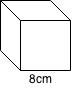# Using exponents to solve problems

### Using exponents to solve problems

In this section, you will try to apply what we learn about exponents in the previous sections to solve word problems such as, calculating the volume of a cube, and more.

#### Lessons

• Introduction
a)
Powers VS. Exponents

• 1.
Write the volume of the cube below as a power. Then, calculate the volume.• 2.
Suppose there are 30 bacteria in a bottle. The number of bacteria doubles every hour. How many will be there after:
a)
1 hour?

b)
7 hours?

c)
n hours?